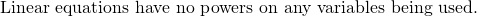# Chapter 2: Linear Equations

The study of linear equations is the study of the foundations of algebra that lead into multiple applications and more advanced mathematics, physics, and engineering fields. Linear equations are used quite frequently in these fields in part because the solutions to complex, non-linear systems can often be well approximated using a linear equation.

Linear equations are equations that can define multiple dimensions. Consider, for instance, the following two equations: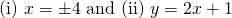Here they are in graphed form: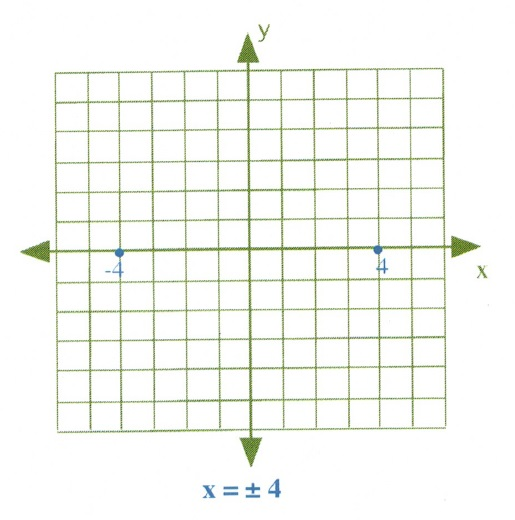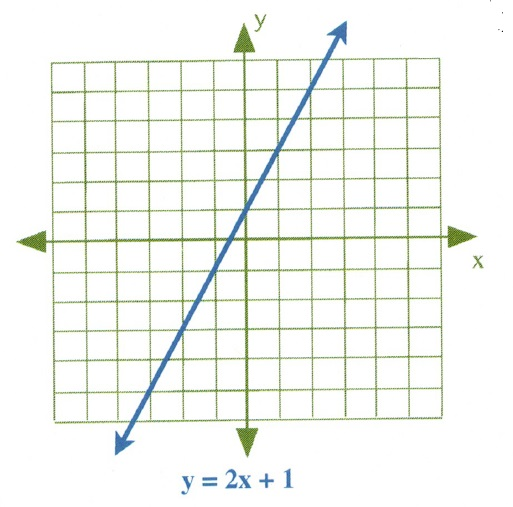Linear equations can also be shown in three dimensions, but that would require time-spaced snapshots to show how a three-dimensional line would change if the fourth dimension of time were to be added.

Fundamental to all linear equations is that the variables being worked with have no powers attached to them. This means that a four-dimensional space-time linear equation could look like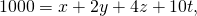but it could not carry any powers, like in the equation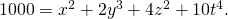Remember: